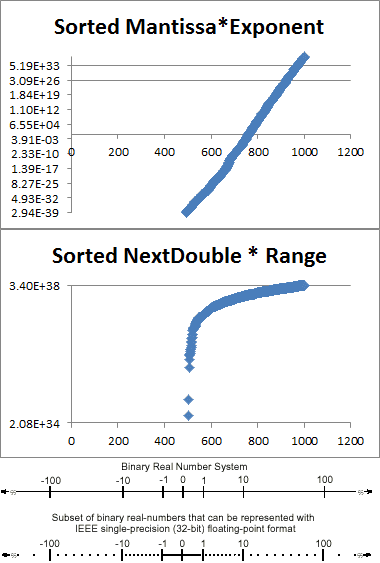# 在C＃中生成随机float的最佳方法

``static float NextFloat(Random random) { double mantissa = (random.NextDouble() * 2.0) - 1.0; double exponent = Math.Pow(2.0, random.Next(-126, 128)); return (float)(mantissa * exponent); }` `

` `static float NextFloat(Random random) { var buffer = new byte; random.NextBytes(buffer); return BitConverter.ToSingle(buffer,0); }` `

` `static float NextFloat(Random random) { // Not a uniform distribution wrt the binary floating-point number line // which makes sense given that NextDouble is uniform from 0.0 to 1.0. // Uniform wrt a continuous number line. // // The range produced by this method is 6.8e38. // // Therefore if NextDouble produces values in the range of 0.0 to 0.1 // 10% of the time, we will only produce numbers less than 1e38 about // 10% of the time, which does not make sense. var result = (random.NextDouble() * (Single.MaxValue - (double)Single.MinValue)) + Single.MinValue; return (float)result; }` `` `// Perform arithmetic in double type to avoid overflowing double range = (double) float.MaxValue - (double) float.MinValue; double sample = rng.NextDouble(); double scaled = (sample * range) + float.MinValue; float f = (float) scaled;` `

` `static float NextFloat(Random random) { (float)(float.MaxValue * 2.0 * (rand.NextDouble()-0.5)); } //inline version float myVal = (float)(float.MaxValue * 2.0 * (rand.NextDouble()-0.5));` `

• 是第二快（见基准）
• 均匀分布

` `static float NextFloat(Random random) { return float.MaxValue * ((rand.Next() / 1073741824.0f) - 1.0f); } //inline version float myVal = (float.MaxValue * ((rand.Next() / 1073741824.0f) - 1.0f));` `

• 是最快的（见基准）
• 是均匀分布的，但是因为Next（）是一个31位的随机值，它只会返回2 ^ 31的值。 （相邻值的50％将具有相同的值）

testing这个页面上的大部分function： （i7，release，没有debugging，2 ^ 28循环）

` ` Sunsetquest1: min: 3.402823E+38 max: -3.402823E+38 time: 3096ms SimonMourier: min: 3.402823E+38 max: -3.402819E+38 time: 14473ms AnthonyPegram:min: 3.402823E+38 max: -3.402823E+38 time: 3191ms JonSkeet: min: 3.402823E+38 max: -3.402823E+38 time: 3186ms Sixlettervar: min: 1.701405E+38 max: -1.701410E+38 time: 19653ms Sunsetquest2: min: 3.402823E+38 max: -3.402823E+38 time: 2930ms` `

` `static float NextFloat(Random random) { double val = random.NextDouble(); // range 0.0 to 1.0 val -= 0.5; // expected range now -0.5 to +0.5 val *= 2; // expected range now -1.0 to +1.0 return float.MaxValue * (float)val; }` `

` `static float NextFloat(Random random) { float f; do { byte[] bytes = new byte; random.NextBytes(bytes); f = BitConverter.ToSingle(bytes, 0); } while (float.IsInfinity(f) || float.IsNaN(f)); return f; }` `